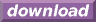#Calcute: freeware calculator

## Hyperbolic Arccotangent (hyperbolic arc-cotangent, hyperbolic inverse cotangent, acoth)

The hyperbolic arc-cotangent is the inverse of the hyperbolic cotangent function. Calcute implements it with the acoth function. The inverse hyperbolic cotangent of a value x is the value y for which the hyperbolic cotangent of y is x. In other words if y = acoth(x) then x = coth(y).

```acoth(1.0087469295621)
2.71828182858
```

The hyperbolic arc-cotangent function is only defined for parameter values lower than -1 and higher than +1. This corresponds to the output domain of the hyperbolic cotangent function which produces no value in the close range [-1,+1].

In some mathematical texts and hand-held calculators, the notation coth-1 is sometimes used to represent the hyperbolic arc-cotangent function. This is an unfortunate notational choice since it could also stand for the multiplicative inverse 1/coth which is a different function.

Yet another term also used to represent the inverse hyperbolic cotangent function is "arccoth".